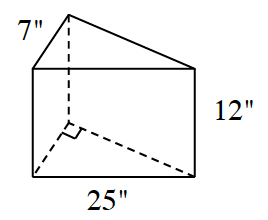### Home > INT2 > Chapter 11 > Lesson 11.2.2 > Problem11-66

11-66.

What is the volume and total surface area of each solid below? Show all work.

1.Use the Pythagorean Theorem to find the missing length of the leg of the triangle.

$V = (\text{base\:area})(\text{height})$

$SA =$ sum of area of the $3$ faces and two bases.

1.For a summary of what you did today, see the math notes box in lesson 11.2.3.

$V = 100\pi m^3\:\:\:\:\:\:\: SA = 90\pi m^2$